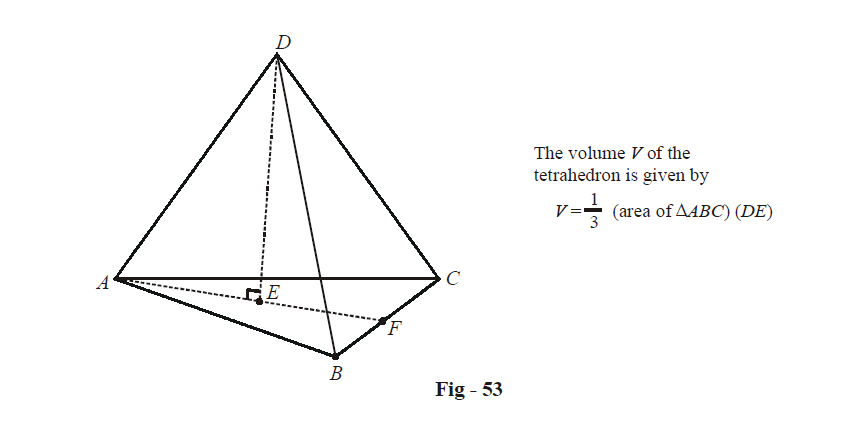# Examples On Vectors Applied To Tetrahedrons Set-2

Go back to  'Vectors and 3-D Geometry'

Learn from the best math teachers and top your exams

• Live one on one classroom and doubt clearing
• Practice worksheets in and after class for conceptual clarity
• Personalized curriculum to keep up with school

Example – 42

Find the angle between any two faces of a regular tetrahedral.

Solution: Refer to Fig - 51 Example 40

The angle between any two faces will obviously equal the angle between the normals to the two faces. Let us find the (acute) angle $$\theta$$ between the normals to the faces OAC and OAB.

\begin{align}&{{\hat n}_{OAC}} = \frac{{\vec c \times \vec a}}{{\left| {\vec c \times \vec a} \right|}} = \frac{{\vec c \times \vec a}}{{\left| {\vec c} \right|\left| {\vec a} \right|\sin \pi /3}} \hfill \\\\& {{\hat n}_{OAB}} = \frac{{\vec a \times \vec b}}{{\left| {\vec a \times \vec b} \right|}} = \frac{{\vec a \times \vec b}}{{\left| {\vec a} \right|\left| {\vec b} \right|\sin \pi /3}} \hfill \\\\& \Rightarrow \quad {{\hat n}_{OAC}} \cdot {{\hat n}_{OAB}} = \frac{{\left( {\vec c \times \vec a} \right) \cdot \left( {\vec a \times \vec b} \right)}}{{{{\left| {\vec a} \right|}^2}{{\left| {\vec b} \right|}^2}{{\sin }^2}\pi /3}}\left( {\because \,\,\,\,\,\left| {\vec a} \right| = \left| {\vec b} \right| = \left| {\vec c} \right|} \right) \hfill \\\\&\qquad\qquad\qquad\qquad= \frac{{\left( {\vec c \cdot \vec a} \right)\left( {\vec a \cdot \vec b} \right) - \left( {\vec c \cdot \vec b} \right)\left( {\vec a \cdot \vec a} \right)}}{{{{\left| {\vec a} \right|}^4}{{\sin }^2}\pi /3}} \hfill \\\\&\qquad\qquad\qquad\qquad= \frac{{\frac{{{{\left| {\vec a} \right|}^2}\left| {\vec b} \right|\left| {\vec c} \right|}}{4} - \frac{{{{\left| {\vec a} \right|}^2}\left| {\vec b} \right|\left| {\vec c} \right|}}{2}}}{{{{\left| {\vec a} \right|}^4}{{\sin }^2}\pi /3}} \hfill \\\\&\qquad\qquad\qquad\qquad = \frac{{ - \frac{1}{4}{{\left| {\vec a} \right|}^4}}}{{{{\left| {\vec a} \right|}^4} \cdot \frac{3}{4}}} = \frac{{ - 1}}{3} \hfill \\\\&\Rightarrow \quad \cos \theta = \frac{1}{3} \hfill \\\\&\Rightarrow\quad \theta ={{\cos }^{-1}}\left( {}^{1}\!\!\diagup\!\!{}_{3}\; \right) \hfill \\ \end{align}

Thus, the angle between any two faces equals \begin{align}{{\cos }^{-1}}\left( \frac{1}{3} \right).\end{align}

Example – 43

The position vectors of the vertices A, B and C of a tetrahedron ABCD are  $$\hat{i}+\hat{j}+\hat{k},\,\,\hat{i}\,and\,\,3\hat{i}$$ respectively. The altitude from the vertex D to the opposite face ABC meets the median line through A of the triangle ABC at E. If AD is 4 units and the volume of the tetrahedron is  \begin{align}\frac{2\sqrt{2}}{3}\end{align} cubic units, find all the possible position vectors of the point E.

Solution:\begin{align} & V=\frac{1}{3}\times \left( \frac{1}{2}\left| \overrightarrow{AB}\times \overrightarrow{AC} \right| \right)\times \left( DE \right) \\\\ & \Rightarrow \quad\frac{2\sqrt{2}}{3}=\frac{\left( DE \right)}{6}\times \left\{ \left| \left( -\hat{j}-\hat{k} \right)\times \left( 2\hat{i}-\hat{j}-\hat{k} \right) \right| \right\} \\\\ & \qquad\qquad\quad=\frac{DE}{6}\times \left\{ \left| -2\hat{j}+2\hat{k} \right| \right\}\\\\&\qquad\qquad\quad =\frac{2\sqrt{2}}{6}\times DE \\\\ & \Rightarrow\; \quad DE\;=\;2 \\ \end{align}

Since $$\Delta ADE$$ is right - angled at E, we have

\begin{align}& AE=\sqrt{A{{D}^{2}}-D{{E}^{2}}} \\\\ &\quad\;\; =\sqrt{16-4} \\\\ & \quad\;\;=2\sqrt{3} \\ \end{align}

It is given that E lies on the median AF. Since F is the mid-point of BC, the position vector of F is $$2\hat{i}.$$

Now, our problem has been reduced to this; we know the position vectors of A and F and we know that $$AE=2\sqrt{3}.$$ We need to find the position vector(s) of E.

Let us suppose E divides AF in the ratio $$\lambda :1.$$ Then the position vector of E is

\begin{align} &\; E\equiv \frac{\lambda (2i)+1\cdot (\hat{i}+\hat{j}+\hat{k})}{1+\lambda } \\\\ &\quad=\left( \frac{1+2\lambda }{1+\lambda } \right)\hat{i}+\left( \frac{1}{1+\lambda } \right)\hat{j}+\left( \frac{1}{1+\lambda } \right)\hat{k}...\left( 1 \right) \\\\ & \Rightarrow \quad \left| \overrightarrow{AE} \right|=\left| \frac{\lambda }{\lambda +1}\left( \hat{i}-\hat{j}-\hat{k} \right) \right| \\\\ &\qquad\qquad\;\; =\left| \frac{\sqrt{3}\lambda }{\lambda +1} \right| \\ \end{align}

Since $$AE=2\sqrt{3},$$ we have

\begin{align}& \quad\;\pm \frac{\sqrt{3}\lambda }{\lambda +1}=2\sqrt{3} \\ & \Rightarrow\quad \frac{\lambda }{\lambda +1}=\pm 2 \\ & \Rightarrow \quad\lambda =-2,\,\,-\frac{2}{3} \\ \end{align}

Using these values of  $$\lambda$$ in (1), the possible position vectors of E are  $$(3\hat{i}-\hat{j}-\hat{k})\,\,and\,\,(-\hat{i}+3\hat{j}+3\hat{k}).$$

Vectors
grade 11 | Questions Set 1
Vectors
Vectors
grade 11 | Questions Set 2
Vectors
Three Dimensional Geometry
grade 11 | Questions Set 2
Three Dimensional Geometry
Three Dimensional Geometry
grade 11 | Questions Set 1
Three Dimensional Geometry
Vectors
grade 11 | Questions Set 1
Vectors
Vectors
grade 11 | Questions Set 2
Vectors